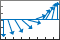# feather

•## 语法

``feather(U,V)``
``feather(Z)``
``feather(___,LineSpec)``
``feather(ax,___)``
``f = feather(___)``

## 说明

``feather(U,V)` 绘制以 x 轴为起点的箭头。使用笛卡尔分量 `U` 和 `V` 指定箭头方向，其中 `U` 表示 x 分量，`V` 表示 y 分量。第 n 个箭头的起始点位于 x 轴上的 n。箭头的数量与 `U` 和 `V` 中的元素数相匹配。`

``feather(Z)` 使用 `Z` 指定的复数值绘制箭头，实部表示 x 分量，虚部表示 y 分量。此语法等效于 `feather(real(Z),imag(Z))`。`

``feather(___,LineSpec)` 设置箭头的线型、标记符号和颜色。`

``feather(ax,___)` 在指定坐标区（而不是当前坐标区）中绘制箭头。`

``f = feather(___)` 返回由包含 `length(U)+1` 个元素的 `Line` 对象组成的向量。前 `length(U)` 个元素表示各个箭头，最后一个元素表示沿 x 轴的水平线。创建绘图后，使用这些 `Line` 对象来控制绘图的外观。`

## 示例

```t = -pi/2:pi/8:pi/2; u = 10*sin(t); v = 10*cos(t); feather(u,v)``````th = -pi/2:pi/16:0; r = 10*ones(size(th)); [u,v] = pol2cart(th,r); feather(u,v)``````Z = [2+3i -1-3i -1+i 2i 3-4i -2-2i -2+4i 0.5-i -3i 1+i]; feather(Z)``````t = -pi/2:pi/8:pi/2; u = 10*sin(t); v = 10*cos(t); feather(u,v,'r')``````t = -pi/2:pi/8:pi/2; u = 10*sin(t); v = 10*cos(t); f = feather(u,v);```

```f1 = f(1); f1.Color = 'r'; f1.LineWidth = 2;``````tiledlayout(1,2) % Left plot ax1 = nexttile; t = 0:pi/8:pi/2; u1 = 10*sin(t); v1 = 10*cos(t); feather(ax1,u1,v1) title(ax1,'Left Plot') % Right plot ax2 = nexttile; u2 = zeros(5,1); v2 = [1 -2 3 -4 5]; feather(ax2,u2,v2) title(ax2,'Right Plot')```## 输入参数

x 分量，指定为标量、向量或矩阵。指定笛卡尔值。要将数据从极坐标转换为笛卡尔坐标，请使用 `pol2cart`

`U` 的大小必须与 `V` 的大小匹配。

y 分量，指定为标量、向量或矩阵。指定笛卡尔值。要将数据从极坐标转换为笛卡尔坐标，请使用 `pol2cart`

`V` 的大小必须与 `U` 的大小匹配。

`"-"`实线`"--"`虚线`":"`点线`"-."`点划线`"o"`圆圈`"+"`加号`"*"`星号`"."``"x"`叉号`"_"`水平线条`"|"`垂直线条`"square"`方形`"diamond"`菱形`"^"`上三角`"v"`下三角`">"`右三角`"<"`左三角`"pentagram"`五角形`"hexagram"`六角形`"red"``"r"``[1 0 0]``"green"``"g"``[0 1 0]``"blue"``"b"``[0 0 1]``"cyan"` `"c"``[0 1 1]``"magenta"``"m"``[1 0 1]``"yellow"``"y"``[1 1 0]``"black"``"k"``[0 0 0]``"white"``"w"``[1 1 1]`